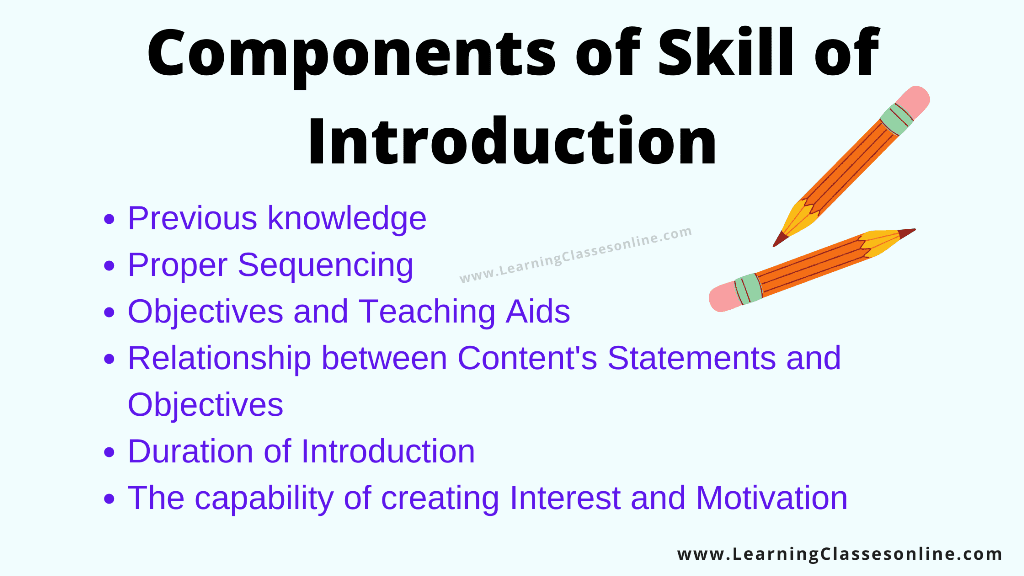Micro Teaching Skill of Introduction | Set Induction

Micro Teaching Skill of Introduction | Set InductionWhat is Micro Teaching Skill of Introduction?

The microteaching skill of the Introduction is also known as the Skill of Set Induction. It is related to the beginning of class teaching. If the beginning of class teaching is effective then the outcomes of the teaching-learning process can be estimated very clearly.

In this Skill the teacher is very active and creative, the teacher has to grab the student's attention in the teaching-learning process,

• He/She creates such an environment that they can start the lesson very effectively.
• For set Induction teachers ask a set of questions to students related to their previous knowledge in order to come with the teaching topic.
• Questions are designed in such a way that the maximum number of students can respond to them.
• This makes students active in class and their interest can be seen by the beginning of the class. Teachers use lots of tricks and tactics for starting the lesson in the class.

The techniques are related to a number of components or the introductory questions are related to its components which are as follows:

Components of Microteaching Skill of Introduction or Set Induction1. Previous knowledge
2. Proper Sequencing
3. Objectives and Teaching Aids
4. Relationship between Content's Statements and Objectives
5. Duration of Introduction
6. The capability of creating Interest and Motivation

Previous knowledge

• During setting the introductory questions teacher should take care of the previous knowledge of students. These questions are very important for starting a new lesson among students.
• Sometimes the teacher starts by showing some objects related to the topic and sometimes asks questions related to previous knowledge.
• The questions should be designed in such a way that maximum students know the answer to them and questions must be interesting so the students give the attention in class.

Proper Sequencing

The ideas, questions, and statements which are made for starting the lesson must have coordination otherwise students can be misleading in the classroom. Questions should be arranged in proper sequencing.

Objectives and Teaching Aids ( TLM )

• Teaching Objectives are very important, the TLM or teaching Aids are designed in order to create interest in classroom teaching so that the students keep attention in class.
• The TLM is always related to the teaching objectives which are previously set.
• Nowadays very creative TLM is being used by teachers for classroom teaching, in doing so all students will keep attention in class and the rate of learning will be enhanced.
• Attractive teaching aids always work well and develop creativity in students.
• By the use of TLM or teaching aids students do not feel monotonous, they learn with joy and motivation.

Relationship between Content's Statements and Objectives

• This is very important for a teacher that there should be a relation between his statement and teaching objectives otherwise students cannot make any judgment about the teaching topic.
• One more thing the teacher's statement must be related to objectives otherwise the teaching topic will not come out properly among students.

Duration of Introduction

The duration of the introduction must not be too short and too long, it must be in such a way that the students take interest and get motivated enough so that a teacher can start the lesson.

The capability of creating Interest and Motivation

• The teacher must have the capability of creating Interest and motivation among students, in short teacher must be a good motivator too. This is a very important point for pupil-teacher or student-teacher who are under training.
• During the Teaching, motivation and a good classroom environment can enhance the rate of learning, this is because students get motivated and then participate in the teaching-learning process.

introduction skill in microteaching ppt

example of introduction skill

introduction skill in microteaching in science

importance of introduction skill

skill of introducing a lesson pdf

skill of introducing a lesson in social science

skill of introduction ppt

importance of introduction skill in teaching

skill of introducing a lesson pdf

skill of introducing a lesson in social science

micro lesson plan on skill of introduction in maths

micro lesson plan on skill of introduction in science

micro teaching skills

skill of introducing a lesson pdf

skill of introducing a lesson components

skill of introducing a lesson ppt

skill of introducing a lesson in social science

skill of introducing a lesson in science

skill of introducing a lesson in mathematicsSimilar Posts

💁Hello Friends, If You Want To Contribute To Help Other Students To Find All The Stuff At A Single Place, So Feel Free To Send Us Your Notes, Assignments, Study Material, Files, Lesson Plan, Paper, PDF Or PPT Etc. - 👉 Upload Here

अगर आप हमारे पाठकों और अन्य छात्रों की मदद करना चाहते हैं। तो बेझिझक अपने नोट्स, असाइनमेंट, अध्ययन सामग्री, फाइलें, पाठ योजना, पेपर, पीडीएफ या पीपीटी आदि हमें भेज सकते है| -👉Share Now

Bcoz Sharing Is Caring😃

1.